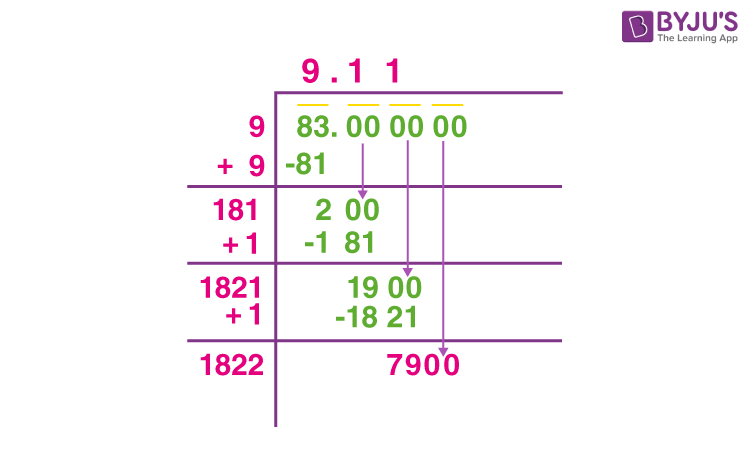# Root 83 simplified

As 83 is not a perfect square, we won’t get the square root of 83 as a whole number.

Now, 92 < 83 < 102

That is, the square root of 83 must lie between 9 and 10.

By long division method of finding the square root:

Step 1: Write 83 as 83.0000 and make pairs of two from right to left.

Step 2: Now take 83, since 9 × 9 = 81 divide 83 by 9 to get the remainder 2

Step 3: Now take down another pair i.e., 00. We now have 200, divide it by 181 to get the remainder 19.

Step 4: Again take down another pair and the above processHence, √83 = 9.11 (approx)(1)(0)